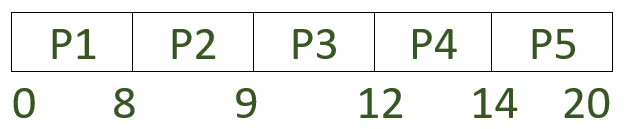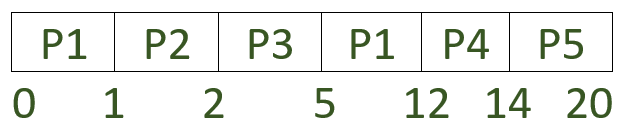GeeksforGeeks App
Open AppBrowser
Continue

# Difference between Preemptive Priority based and Non-preemptive Priority based CPU scheduling algorithms

Prerequisite – CPU Scheduling
Priority Scheduling :
In priority scheduling, each process has a priority which is an integer value assigned to it.
The smallest integer is considered as the highest priority and the largest integer is considered as the lowest priority. The process with the highest priority gets the CPU first.

In rare systems, the largest number might be treated as the highest priority also so it all depends on the implementation.

If priorities are internally defined then some measurable quantities such as time limits, memory requirements, the number of open files and the ratio of average I/O burst to average CPU burst are used to compute priorities.
External priorities are assigned on the basis of factors such as the importance of process, the type and amount of funds been paid for computer use, the department sponsoring of the work, etc.

Preemptive and non-preemptive SJF is a priority scheduling where priority is the shortest execution time of job. In this algorithm, low priority processes may never execute. This is called starvation.

The solution to this starvation problem is aging. In aging, as time progresses, increase the priority of the process so that the lowest priority process gets converted to the highest priority gradually.

1. Priority Preemptive Scheduling :
Sometimes it is important to execute higher priority tasks immediately even when a task is currently being executed. For example, when a phone call is received, the CPU is immediately assigned to this task even if some other application is currently being used. This is because the incoming phone call has a higher priority than other tasks. This is a perfect example of priority preemptive scheduling. If a task with higher priority than the current task being executed arrives then the control of the CPU is taken from the current task and given to the higher priority task.

2. Priority Non-Preemptive Scheduling :
Unlike priority preemptive scheduling, even if a task with higher priority does arrive, it has to wait for the current task to release the CPU before it can be executed. It is often used in various hardware procedures such as timers, etc.

Note:
If all the processes arrive at the same time then priority preemptive scheduling and priority non-preemptive scheduling work in the same manner.

Now let’s do a comparative study of preemptive priority scheduling and non-preemptive priority scheduling.

Example:

Let’s try and solve this problem using both algorithms to do a comparative study.

1. Priority Non-preemptive scheduling :
The Gantt chart will look like:Average waiting time (AWT),

```= ((0-0) + (8-1) + (9-2) + (12-3) + (14-4)) / 5
= 33 / 5
= 6.6 ```

Average turnaround time (TAT),

```= ((8-0) + (9-1) + (12-2) + (14-3) + (20-4)) / 4
= 53 / 5
= 10.6 ```

2. Priority Preemptive scheduling :
The Gantt chart will look like:Average waiting time (AWT),

```= ((5-1) + (1-1) + (2-2) + (12-3) + (14-4)) / 5
= 23/5
= 4.6 ```

Average turnaround time (TAT),

```= ((12-0) + (2-1) + (5-2) + (14-3) + (20-4)) / 5
= 43 / 5
= 8.6 ```
My Personal Notes arrow_drop_up